Section 21.4. Fine-Tuning Pivot Table Calculations

21.4. Fine-Tuning Pivot Table Calculations

As you saw earlier, when you add a field to the Values box, Excel guesses what calculation you want to perform. In most cases, it assumes you want to perform a sum operation that totals up all the values in the field. However, this calculation isn't always the right one. Consider the sales summary pivot table that you've been exploring in this chapter. Although it makes sense to examine the total units sold, you may be just as interested in the maximum, minimum, or average order size , or the order count (the number of times the product was ordered, without considering the number of units in each order).

Fortunately, Excel makes it easy to change the type of calculation you're using. And as you'll see in the following sections, you can even perform more than one calculation in the same pivot table, and throw your custom formulas into the mix.

 GEM IN THE ROUGH Avoiding Slow Refreshes If your pivot table involves a huge amount of information, and if you plan to use multiple levels of grouping, you may not like Excel's standard behavior. Every time you drag a field to a box in the PivotTable Field List pane, Excel regenerates the pivot table with your new settings. This refresh takes a bit of time, and it can make designing a complex pivot table seem just a little sluggish . If you're tired of waiting, Excel has a solution. Before you make any changes to your pivot table, switch on the Defer Layout Update setting, which appears at the bottom of the PivotTable Field List pane. While the Defer Layout Update is on, Excel doesn't refresh your pivot table as you build or change it. If you're a fast mover and you're dealing with a massively large pivot table, this lets you work much faster. When you're finished making your changes (or you just want to see what the pivot table looks like so far), click the Update button to perform a one-time refresh. Or, turn off the Defer Layout Setting to go back to normal, and let Excel refresh the pivot table automatically. Of course, there is one disadvantage to using Defer Layout Update. You don't see the effects of your changes until you're finished. As a result, the Defer Layout Update setting is a great tool for pivot table pros, but you'll probably want to stay away from it while you're learning.

21.4.1. Changing the Type of Calculation

To modify the calculation that the pivot table performs , follow these steps:

1. Find the appropriate field in the Values box of the PivotTable Field List pane. Click the drop-down arrow, and then choose Value Field Settings .

If you want to change the current operation, which sums together the Quantity value for each row in a group , then click the "Sum of Quantity" item in the Values box.

When you do, the Value Field Settings dialog box appears (Figure 21-15).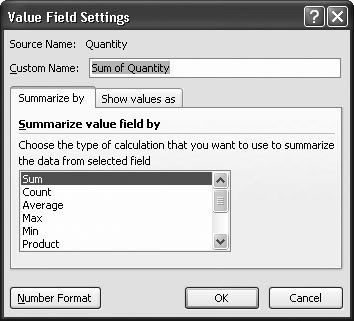Figure 21-15. Using the "Summarize by" tab, you can choose the subtotaling calculation you want to perform. You can use counts, averages, sums, or find the maximum value, minimum value, or standard deviation. The "Show Values as" tab lets you configure more complex calculations that compare fields (like differences, percentages, and so on) or calculate running totals.

2. In the "Summarize by" tab, choose a different option from the list .

Choose Count to add up the number of different orders for a product, or choose Average to calculate the average order size.

3. If you want to define a different number format to display the summary information, click the Number Format button, choose a new format, and then click OK .

When you click Number Format, Excel shows a slimmed-down version of the Format Cells dialog box, which only includes the Number tab (Section 5.1.1). You can use this tab to change the number of decimal places, get a currency symbol, and so on.

4. Click OK to close the Value Field Settings dialog box .

Excel refreshes the pivot table with the new information.

 POWER USERS' CLINIC Adding Multiple Values As you've seen, you can add more than one field to the Row Labels and Column Labels boxes. You can use the same technique to add multiple fields to the Values box. When you do, each field is calculated and shown on the pivot table, in a separate column (as shown in Figure 21-16). You could decide to show the total for the Quantity field and the average of the Unit Price field. To do so, drag both fields into the Values box. Then, follow the steps on this page to configure the type of calculation that's performed for each field. You can also use this technique to perform multiple calculations with the same field. If you want to average and total the Quantity field, drag the Quantity field into the Values box twice. You'll end up with two separate items, which you can configure separately.

Tip: These column headings that are used for data values can use up some valuable space. To get narrower columns , you can apply a shorter custom column name. To do so, click the field in the Values box, and then choose Value Field Settings. In the Value Field Settings dialog box, enter a new field name in the Custom Name box.

21.4.2. Adding a Calculated Field

Using the pivot tables you've learned about so far, you can perform a variety of operations on any field, including averages, sums, and other preset calculations. But what if you want to branch out a little further and create a custom calculation? You could alter the source data and add a new column containing your calculation, and then recreate the pivot table. But Excel provides a much easier option by letting you define a calculated field.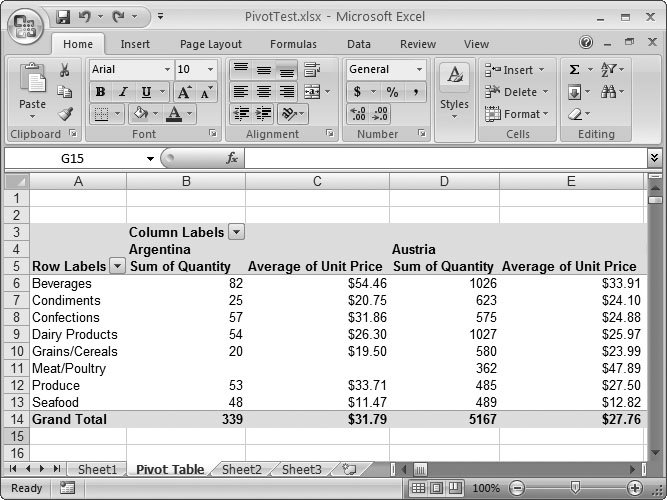Figure 21-16. When your pivot table contains multiple values, they appear in different columns with headings like "Sum of Quantity" and "Average of Unit Price."

The following steps show how to create a calculated field within a pivot table. Using these steps, you can modify a pivot table that shows a list of orders so that it totals the amount of revenue generated instead of just adding up the number of units shipped.

1. Move anywhere inside the pivot table .

2. Choose PivotTable Tools Options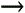ToolsFormulasCalculated Field .

The Insert Calculated Field dialog box appears (as shown in Figure 21-17).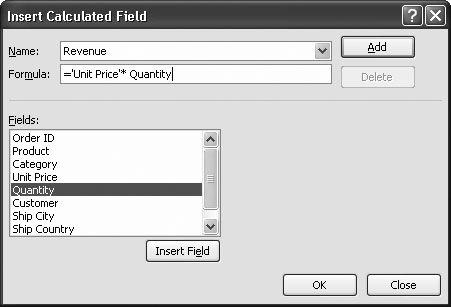Figure 21-17. Here, Excel creates a new field named Revenue to calculate how much money is being made on any given order. To arrive at the total revenue, the Unit Price field is multiplied by the Quantity field.

3. Enter a name for the new field in the Name text box .

In this case, the new name is Revenue.

4. Enter the formula this field uses in the Formula text box .

(This is a standard Excel formula that modifies one or more of the other fields, which are provided in the Fields list.)

Your formula can use Excel's built-in functions, or you can enter a formula that alters or combines one or more of the fields that are in the Fields list. The new Revenue field calculates the total revenue generated by multiplying the price of the item by the quantity purchased. It uses the following formula:

='Unit Price'* Quantity.

Note that field names that include spaces or special characters , like Unit Price, need to be enclosed in apostrophes. If you're in any doubt, double-click the field name in the list to have Excel insert it into the Formula text box with apostrophes if they're necessary.

5. Click OK .

Excel automatically adds the calculated field to the PivotTable Field List pane and then inserts it in the Values box, so that it appears in the pivot table (Figure 21-18).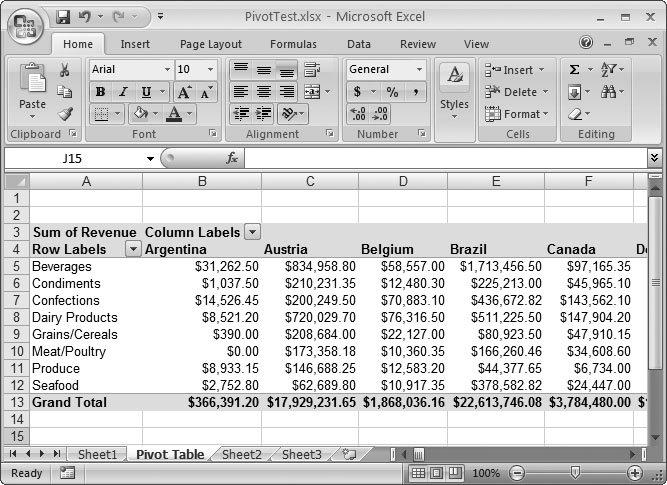Figure 21-18. The worksheet now shows the total revenue by category and customer country.

As with any other numeric field, Excel assumes you want to perform a sum calculation that totals up your formula for every row. If you want to perform a different calculation, you can customize your field as described in the previous section.

Note: Even if you drag your custom field out of the Values box to remove it from the pivot table, it remains in the field list. If you want, you can drag it back to the Values box later on.Excel 2007[c] The Missing Manual
ISBN: 596527594
EAN: N/A
Year: 2007
Pages: 173